Polygon triangulationDownload Polygon programDownload complete Delphi-7 project

Introduction

Triangulation is necessary if the polygon has to be colored or when the area has to be calculated.
The painting or calculations may then be performed on the individual triangles, instead of the complete
and sometimes complicated shape of the polygon.

Polygons may appear in different shapes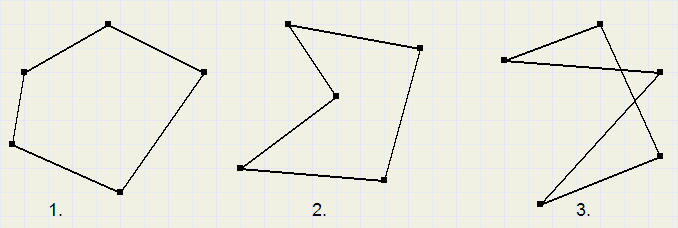1. is a convex polygon, each angle is less than 180 degrees.
2. has an "inside" angle, bigger than 180 degrees.
3. has intersecting edges.

In this application type 3. is illegal. The program will raise an error message.

types 1. and 2. , whatever complicated, can be broken down into triangles.
These individual triangles then may be colored or the areas may be summed.

A polygon is made up of sequential points interconnected by lines.
There is an area inside- and an area outside the polygon.
When decomposing the polygon into triangles, the biggest problem is to classify an angle as "inside" or "outside".
In case 1. before, each angle is "outside" and 3 successive points always are angles of a triangle
which is inside and part of the polygon.
Triangulation may also be accomplished by drawing lines from one point to all others.
In the case of polygon 2. however, the "inside" angle must be recognized to avoid coloring.

To distinguish "outer"- and "inner" angles I use some basic vector geometry.

Outer- and Inner angles

A line (edge) has a starting and ending point.
In mathematics it is called a vector, because more than one number is required to describe it.
(2 numbers, in 2D geometry).
The next figure shows line l with vector AB.
An arbitrary point on line l may be characterized by a single number, I call this factor f.
Point A, the starting point, corresponds with f = 0. Point B, the ending point, corresponds with f = 1.
Right of B (the forward extension of AB) has points that correspond to f > 1.
Left of A (the backward extension of AB) has points that correspond to f < 0.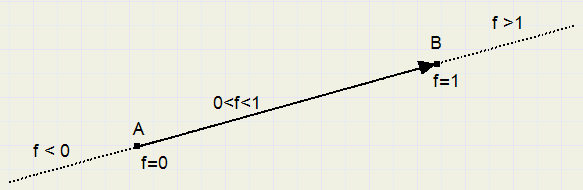In case of two vectors (red and blue) there is an intersecting point S.
Since S is on both the red and the blue vector, we may calculate the f values (f1 for red, f2 for blue) for S.
f1 and f2 give an indication of the relative position of the vectors.Red and blue may be parallel or coincide. In that case there is no intersection.
A flag fvalid is set false in this case.
Using this vector geometry we are able to distinguish between inner- and outer angles in
case of simple polygons. Look at the next figure: we examine angle B.

In case 1. B is an outer angle because the forward extension of AB does not intersect other vectors.
In case 2. the forward extension of AB intersects DE in point S, so B is an inner angle.
In case 1. we may color triangle ABC, in case 2. we may not.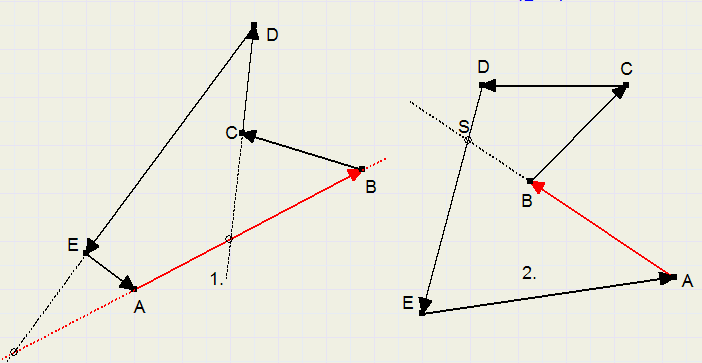However.... look at the following figure: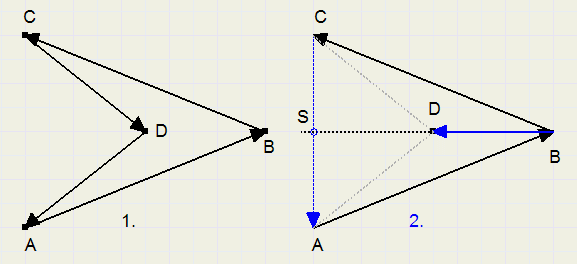1. shows polygon ABCD. Point B is an outer angle, but triangle ABC may not be colored
because there is a point (D) inside triangle ABC.
2. shows how to recognize this situation.
Construct vectors CA and BD and investigate if the forward extension of BD intersects CA .
Of course this must be repeated for all points (D) other than A,B,C.
For simple polygons as bars or arrows, this algorithm is fine.

The complete algorithm

Below is a more complex polygon for which the so far established algorithm does not work.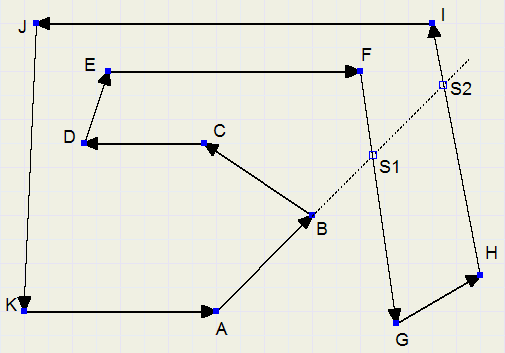Extended vector AB intersects FG in S1 (and HI in S2) but angle B still is an outer angle.
So, the "outer-angle" test needs reconsideration.
We notice that the extension of AB intersects two other vectors, not one.
That is already an important step toward the solution of this case.
Odd number of intersections : inner-angle, even number of intersections: outer angle.
But there still is a problem to be solved, have a look: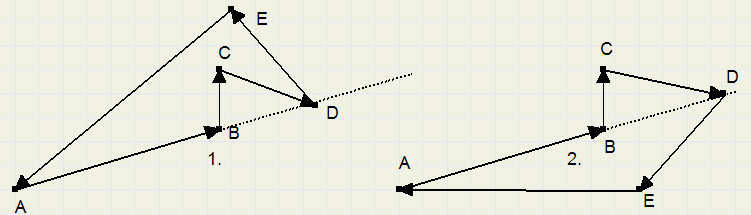The extension of AB intersects two other vectors (CD and DE) in exactly their start- and ending point D.
In case 1. B is an outer-angle, but in case 2. B is not.
In case 1. ABC may be colored, in case 2. not.
What to do? I present the following solution after having evaluated all possibilities: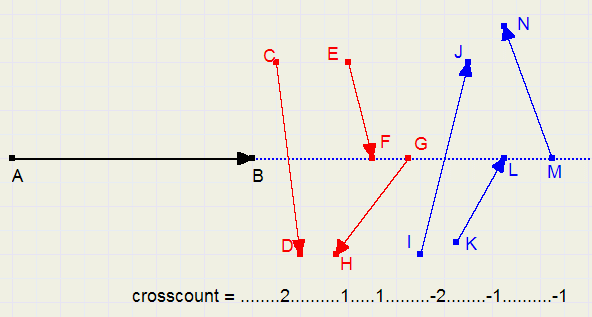Position yoursef at point A en look at B and further.
Vectors intersect the extension of AB and they may cross to the right (CD,EF,GH) or to the left (IJ,KL,MN).
Start- or ending points may be on (extended) AB, or not.
For all of these cases we apply a score: the crosscount. See figure. The simple rule for an outer-angle now is:
Outer-angles have a crosscount sum of zero
Note: a parallel vector has a crosscount of zero. Nothing is added to the sum of crosscounts.

Please refer to the next illustration with crosscount values for vector AB :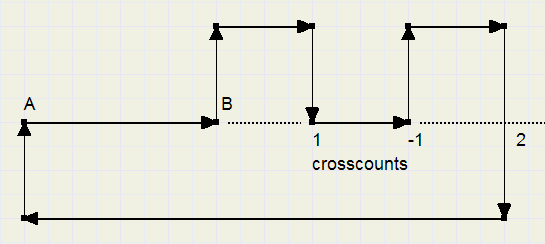The sum for all AB crosscounts is 1 -1 + 2 = 2.
This is not equal to zero so, B is not an outside-angle.

Remains a method to recognize a positive or negative crosscount.
It is obvious to measure the angle between the vectors for this.
Shift the vectors parallel until their starting points coincide.

We notice RIGHT................0 < angle < 180 degrees ..............or
-360 < angle < -180 degrees

(relative to AB)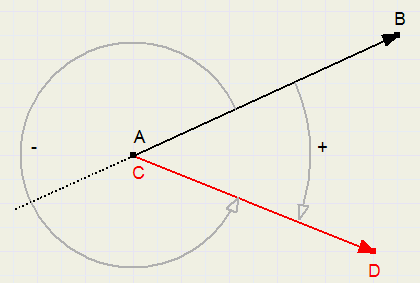The angle between two vectors is the difference of their directions.

Where pi = 3.14......the ratio of the circumference and diameter of a circle.
The directions are :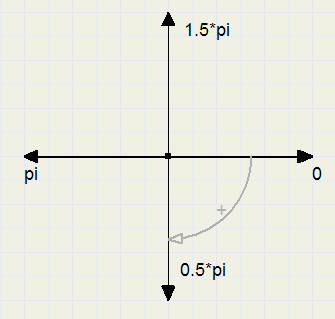This completes the algorithm. Time to descent into the implementation details.

Data structures

```const maxpolypoint = 100;//maximal number of points allowed
```
points are defined in array points:
```var points : array[1..maxpolypoint] of Tpoint;
pcount : byte; //number of valid points in array points[ ]
```
The first and last points in the array must be the same : the polygon is "closed".
Using array points, the vectorlist is generated.
```type Tvector = record
x,y : longInt;   //coordinates of starting point
dx,dy : longInt; //hor., vert length
dir : double;    //direction in radians
end;

var vectorlist : array[1..maxpolypoint] of TVector;
vcount : byte;  //number of vectors
```
From the vectorlist the trianglelist is made.
```type Ttriangle = record
ax,ay,bx,by,cx,cy : longInt;//(x,y) of A,B,C
end;

var trianglelist : array[1..maxpolypoint] of Ttriangle;
tcount : byte;//number of entries in trianglelist
```
Coding a vector: (screen coordinates)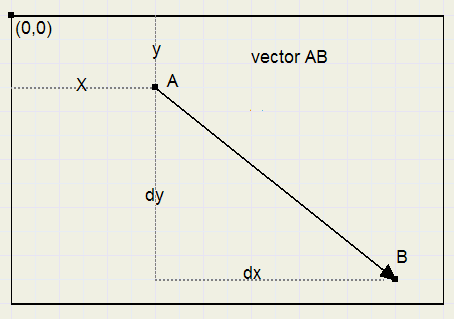The vectorlist is brooken down (vectors removed) as the trianglelist is build.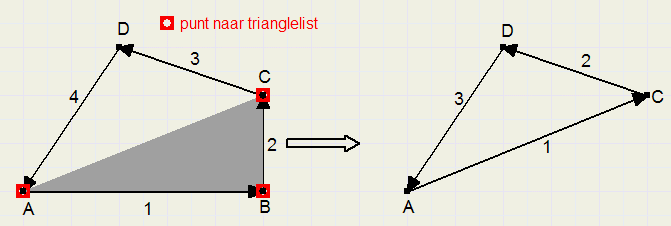The red points are enterd in the triangle list.
See figure: vector 1 is replaced by the sum (AC) of vectors 1. and 2. and points A,B,C are entered in the trianglelist.

The trianglelist is the final table: from here individual triangles may be colored or the area be calculated.
The area of a triangle, given the coordinates of the angles, is calculated using the lemma of Heron:
area = sqrt(s*(s-a)*(s-b)*(s-c)).
where a,b,c are the lengths of the edges and s = 0.5*(a+b+c) , half the perimeter.
a,b,c may simply be calculated using the Pythagoras lemma.
For the proof of the Heron lemma please look here

The structure of the polygon project

All type definitions, variables, functions and procedures for the polygons are housed in unit poly_unit.
calls:
```function polyArea(pts:pointer; n:byte) : double;//area of polygon
```
pts points to points, n is the number of valid points in points[ ];
This function returns the area and draws the polygon on the previously selected canvas.
```procedure setCanvas(cvs : Tcanvas);             //select canvas
procedure setpencolor(pc : longInt);            //pen color
procedure setBGcolor(bgc : longInt);            //background color
procedure setBG(BGmode : boolean);              //BG true/false
```
BG true: the background is painted. BG false: only the lines and points are painted.
```procedure geoClear;                             //debug purposes
```
initialize all arrays.
```procedure setShowTriangles(trMode : boolean);   //triangles on/off
```
trMode = true: draw the triangles, will be used only for test purposes.
```function polyResultMessage : string;            //message of code
```
Global variable polyResultCode is the measure for good processing.
Zero is OK, nonzero indicates an error.
Function PolyResultmessage returns a string describing the polyResultCode.

Global variables in poly_unit:
```var polyresultcode : byte;
polyTime : longInt;
```
polyResultcode = 0 if eror free processing
polyTime is the process time in microseconds.
unit Clock_unit contains procedures to measure execution times using the CPU cycles clock.

To design and test the algorithm a so called exerciser is build in form1/unit1;
This allows generation and modification of polygons. Results are visualised.

The exerciser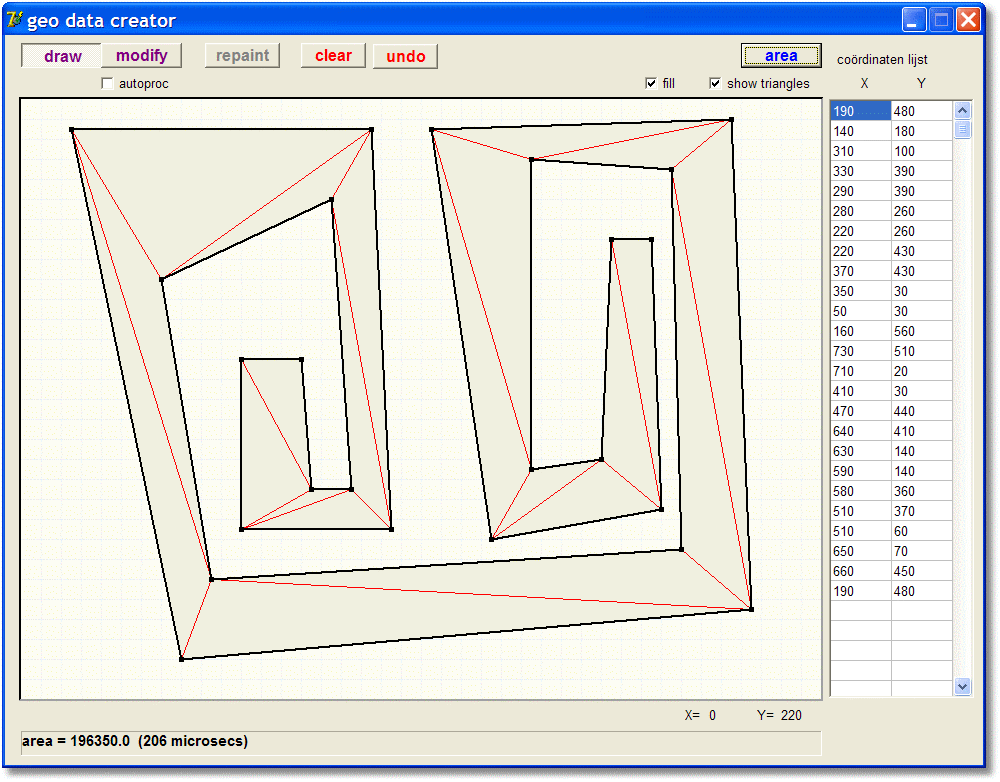The exerciser operates in draw- of modify mode.
draw: use mouse to draw lines. Undo or backspace removes the last line.
modify: position mousepointer on angle, press mousbutton and move point to new position.
Press to increment mousepointer pixel by pixel. Else use steps of 10 pixels.

Checkbox "autoproc" : draw and calculate polygon after each modification.
Checkbox "fill" : paint background in polygon.
Checkbox "show triangles" : draw individual triangles of polygon.
BitBtn "Area" : calculate area and draw polygon.

The poly_unit

This unit only uses the clock_unit, to measure processing times.
Therefore, the poly_unit and the clock_unit may simply be added to a Delphi project
of choice to implement polygon area calculation.
Below is the function that calculates the area:
```function  polyArea(pts:pointer; n:byte) : double;
//calculate area, draw polygon
var t1,t2 : Int64;
begin
getCPUticks(t1);          //clock cycles since power on
polyresultcode := 0;
pp := Ppoints(pts);       //pointer to points
pcount := n;
checkpoints;              //check closed, sufficient points
if polyresultcode <> 0 then exit;

buildvectorlist;
checkvectors;             //crossing edges, duplicate points
if polyresultcode <> 0 then exit;

buildtrianglelist;
if polyresultcode <> 0 then exit;

trianglesums;             //summarize area of triangles
result := area;           //later version: result = area / 400
getCPUticks(t2);
polyTime := ProcTime(t2-t1);
drawpoly;                 //draw polygon on selected canvas
end;
```
There are some "low-level" procedures to attack the vectors.
Most calculations are performed by vrsect, which calculates the f1 and f2 factors of the intersection of two vectors.
(see appendix for the math)
```procedure vrsect(const v1,v2 : TVector);
//calculate intersection of vectors v1,v2
//line1 = (v1.x1,v1.y1) +f1*(v1.dx,v1.dy)
//line2 = (v2.x1,v2.y1) +f2*(v2.dx,v2.dy)
//return f1,f2,fvalid
var d,vx,vy : double;
begin
d := v1.dx*v2.dy - v1.dy*v2.dx;//discriminant
if d = 0 then begin
fvalid := false; exit;
end;
fvalid := true;
vx := v2.x - v1.x;
vy := v2.y - v1.y;
f1 := (vx*v2.dy - vy*v2.dx)/d;
f2 := (vx*v1.dy - vy*v1.dx)/d;
end;
```
Other low-level procedures are:
```function outward(vnr : word) : boolean; //return true if  vectorlist[vnr] points outward
function VDir(deltaX,deltaY : double) : double; //return direction of vector in radians
function Empty3(i1,i2,i3 : word) : boolean;//check for no point (= true) inside triangle i1,i2,i3
```
i1,i2,i3 are sequential indices to the vectorlist[ ]
Of i3, only the vectorlist[i3] starting point is used.
For more details please refer to the source code.

Some hi-level procedures are:
```procedure checkpoints;      //test for sufficient points and closed polygon
procedure buildVectorlist; //Builds vectorlist from array points[ ]
procedure checkvectors;    //Tests for intersecting edges en duplicate points
procedure buildTriangleList;     //builds trianglelist from vectorlist
procedure TriangleSums;   //sums areas of all triangles
```

Note: the bitmap has 20 * 20 pixel squares.
Areas are measured in squares. (later versions)

Drawing procedures are:
```procedure geoTriColor(const tr:Ttriangle;brushcol:LongInt);
```
Draw the background of a triangle, using horizontal lines.Angles are first sorted to ascending values of y.
```procedure drawline(p1,p2 : Tpoint);//Draw line from point p1 to p2.
procedure drawpoint(p : Tpoint);  //draw point p
```

Appendix 1

An introduction to the vector geometry as used in this algorithm.
In plane geometry, a vector simply is a line with length and direction.

notation:
Please refer to the figure below with vectors AB an BC
A B =  ­ d x1 ­ d y1
.............. B C =  ­ d x2 ­ d y2

the sum of two vectors
A B + B C =  ­ d x1 + d x2 ­ d y1 + d y2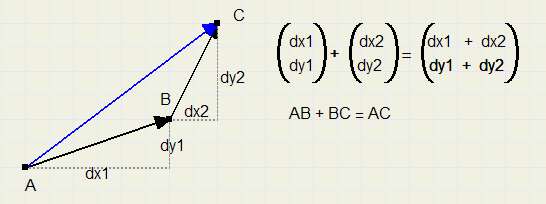multiplication of a vector (enlarge, reduce)
f  ­ d x1 ­ d y1
=  ­ f.d x1 ­ f.d y1
In the figure below, AB is multiplied by 1.5 and also by -0.5
Note : for negative f the vector changes direction.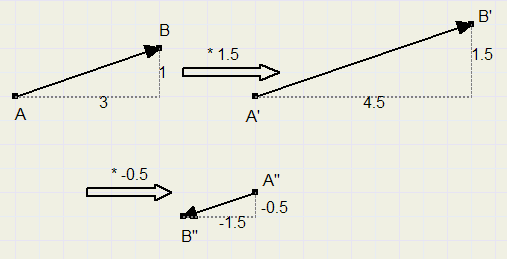The vector equation of a line (normal coordinates, not screen)For an arbitrairy point
 ­ x ­ y
on a line with vector
 ­ d x1 ­ d y1
we may write:
 ­ x ­ y
=  ­ x1 ­ y1
+ f  ­ d x1 ­ d y1
Note: f = 0 for point A, the starting point of the vector.
f = 1 for B, the end of the vector.
For 0 < f < 1 a point is located between A and B.
If f > 1 the point is situated on the forward extension of AB.
If f < 0 then a point is on the backward extension (left of A in this case) of AB.

The intersection of two lines:
line1:  ­ x ­ y
=  ­ x1 ­ y1
+ f1  ­ d x1 ­ d y1

line2:  ­ x ­ y
=  ­ x2 ­ y2
+ f2  ­ d x2 ­ d y2
The only unknowns are the factors f1 anf f2.
To know f1 and f2, so the position of the intersection, we have to solve following set of equations:
x1 + f1*dx1 = x2 + f2*dx2
y1 + f1*dy1 = y2 + f2*dy2
To solve the equations we multiply row 1 by dy2 and row 2 by dx2 :
dy2(x1 + f1*dx1) = x2.dy2 + f2*dx2.dy2 dx2(y1 + f1*dy1) = y2.dx2 + f2*dx2.dy2
Now subtract row 2. from row 1.
dy2(x1 + f1*dx1) - dx2(y1 + f1*dy1) = x2.dy2 - y2.dx2 ........or:
x1.dy2 + f1*dx1.dy2 - y1.dx2 - f1*dx2.dy1 = x2.dy2 - y2.dx2 ............or:
f1(dx1.dy2 - dx2.dy1) = (x2-x1)*dy2 - (y2-y1)*dx2
let
d = dx1.dy2 - dx2.dy1.......and
vx = x2 - x1 .........and
vy = y2 - y1.........then

f1 =  v x.d y2 − v y.d x2 d

and similar
f2 =  v x.d y1 − v y.d x1 d
For d = 0 the vectors coincide or are parallel.
In this case flag fvalid = false indicating there is no intersection point.

Appendix 2

The direction of a vector:

The arctangent function is used to get the direction of a vector.
direction = arctan
æ d y d x
ö
­­
èø
And again we realise that nice applications can be made using only simple math.